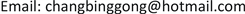1. 引言

1994年，Dickey等人在《科学》杂志上发表论文，表明月亮远离地球的速度是每年3.82 ± 0.07厘米  。

2. 时空阶梯理论简介

F 1 = q ( E 1 + v 1 × B )

F = m ( E + v × Q )

v 1 = H 0 D

v 2 = R Q sin θ

3. 按照哈勃定律计算

The theoretical calculation distance of the eight planets away from the su

The actual observation distance of the eight planets away from the su

4. 经验公式的建立

v = Q D

v是行星(地球等)膨胀的速度，或者卫星(月亮等)膨胀的速度，Q是气感应强度，D是行星到太阳的距离，或者月亮到地球的距离。

k 1 v = Q D ( c v 1 )

v = H 0 D k 1 ( c v 1 ) 2

v = H 0 D k 4 ( c v 1 ) 2

5. 验证经验公式

Average distance from the eight planets to the sun and average orbital spee

Distance of eight planets away from the sun calculated using only one expansion coefficien

Expansion coefficient calculated according to the actual distanc

Comparison between theoretical expansion coefficient and actual expansion coefficien

The difference between theory and actual distanc

v = H 0 D k 4 ( c v 1 ) 2 , k = 306.697266806281 e ( n π 18 ) , ( n = 1 , 2 , 3 , 4 , 5 , 6 , 7 , 8 , ⋯ )

6. 对经验公式的初步解释

7. 精细结构常数的意义

e是基本电荷，

ε 0 是真空电容率，

ℏ 是约化普朗克常数，是普朗克常数，

c是光速。

“狄拉克方程认为光谱的精细结构是由电子的自旋–轨道作用引起的，是一种相对论效应”。相对论效应，在时空阶梯理论看来，就是给形而下时空一个划了一个界限，就是光速和光速以下的是形而下时空，形而下时空不可能超光速，而且形而下时空是收缩的，收缩是是逐渐增强的。狄拉克方程具有更丰富的形而下时空阶梯的内容，所以，狄拉克方程可以描述更丰富的粒子和粒子的自旋  。

F = m c 2 r c n v 1 v 2 v 3 ⋯ v n

1) 原子的精细结构常数变大，说明宇宙膨胀。

2) 原子的精细结构常数变小，说明宇宙收缩。

3) 原子的精细结构常数不变，说明宇宙处于动态平衡状态。就是说，宇宙既不膨胀，也不收缩，或者说宇宙既膨胀又收缩，但是，膨胀和收缩处于动态平衡状态。

8. 宇宙以等角螺线的方式展开

9. 总结